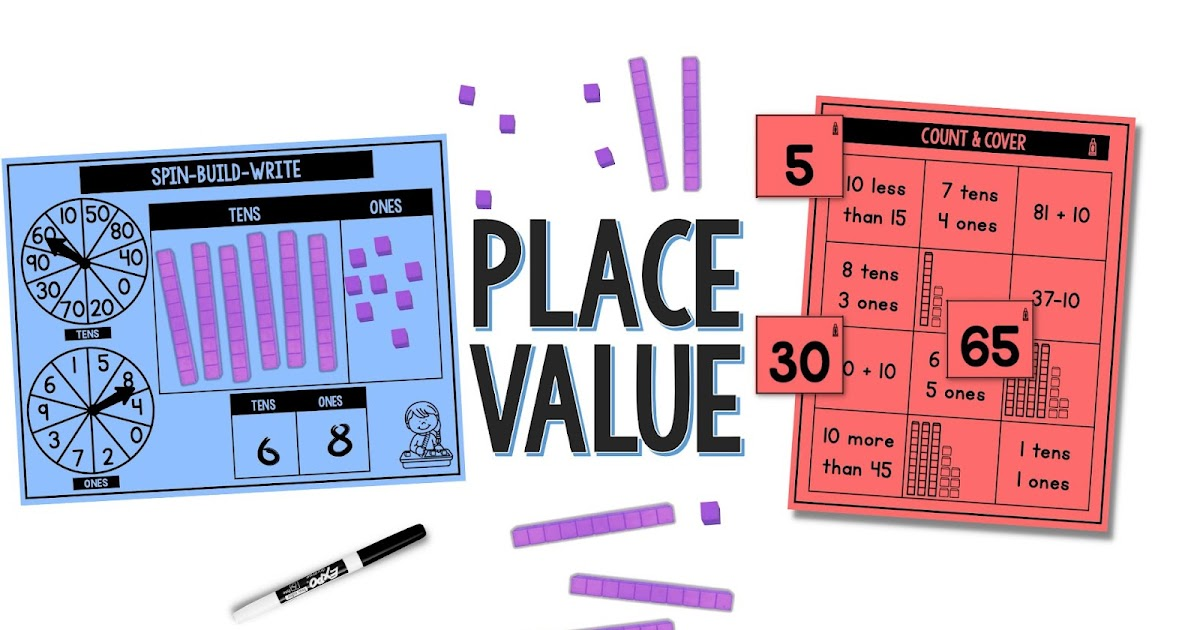Teaching

# Using Base Ten Blocks to Place Value to Kindergarten and First GradeTeaching place value in kindergarten or first grade can be tricky. Place value is a difficult concept for students to master if they do not have a good foundation of number sense.

Place value is one of the most fundamental concepts in mathematics. It helps students understand numbers as collections of units (ones, tens, and hundreds) It can also help students develop an understanding of fractions. Students need to master place value before learning multiplication and division.

Starting in kindergarten “Numbers and Operations in Base Ten” is a math standard that continues through fifth grade. Kindergarten students begin to learn about place value by decomposing numbers into 10 and some ones. In kindergarten students are expected to add and subtract fluently within five.

It is important that students understand the value of digits. This will help them when they are composing and decomposing numbers. First grade is when students begin to explore larger numbers and the concept of place value through decomposing numbers to 100. As students move up in grade levels, the expectation changes all the way to 1000.

#### USING BASE TEN BLOCKS

Base Ten Blocks provide students with a hands-on way to learn place value, number concepts, operations, measurement, and much more! Using base ten manipulative blocks help students physically represent what they’re learning. Through the use of base ten blocks, students can develop a deeper understanding of the meaning of each concept. As students are working and building number combinations in base ten, they are also developing skills needed for the concept of regrouping

#### GAMES AND ACTIVITIES FOR PLACE VALUE

Giving students hands-on practice to work with base ten blocks is crucial. These base ten block activities let students explore while they are learning.